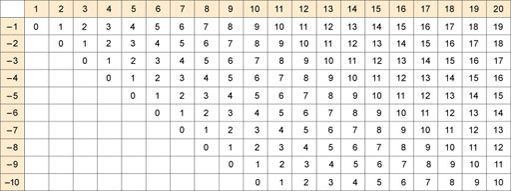Up to now only those points with positive or zero coordinates have been considered. But the system can be made to cope with points involving negative coordinates, such as (−2, 3) or (−2, −3). Just as a number line can be extended to deal with negative numbers, the x-axis and y-axis can be extended to deal with negative coordinates.

[Image_Link]https://www.open.edu/openlearn/ocw/pluginfile.php/94235/mod_oucont
Author(s): The Open University

## Activity 16

Write down the coordinates of A and B.

[Image_Link]https://www.open.edu/openlearn/ocw/pluginfile.php/94235/mod_oucontent/oucont
Author(s): The Open University

For many towns and cities, an individual book of street maps called an A to Z has been produced. You can look up the name of a street in the index, and it will give you the page number of the map that contains the street, plus the grid reference square for the street. There are different conventions for these grid references. You may have met several of these.

## Activity 10

This table categorises Tom's activities for the day.

Activity<
Author(s): The Open University

Pie charts are representations that make it easy to compare proportions: in particular, they allow quick identification of very large proportions and very small proportions. They are generally based on large sets of data.

The pie chart below summarises the average weekly expenditure by a sample of families on food and drink. The whole circle represents 100% of the expenditure. The circle is then divided into ‘segments’, and the area of each segment represents a fraction or pe
Author(s): The Open University

## Activity 7

Tables often give information in percentages. The table below indicates how the size of households in Great Britain changed over a period of nearly 30 years.

Number of people in household1961 (%)1971 (%)1981 (%)1991 (%)
1Author(s): The Open University

## Activity 6

The table below indicates the cooling rate of tea in a teapot.

Time/mins
Author(s): The Open University

Experiments or surveys usually generate a lot of information from which it is possible to draw conclusions. Such information is called data. Data are often presented in newspapers or books.

One convenient way to present data is in a table. For instance, the nutrition panel on the back of a food packet:

### Nutrition Information

Author(s): The Open University

## Activity 1

Grateful acknowledgement is made to the following sources for permission to reproduce material in this course:

The content is taken from an activity written by Marion Hall for students taking courses in Health and Social Care, in particular those studying K101 An Introduction to Health and Social Care. The original activity is one of a set of skills activities made available to all HSC students via the HSC Resource Bank.

Except for third party materials and otherwise stated (see
Author(s): The Open University## Study another free course

There are more than 800 courses on OpenLearn for you t
Author(s): The Open University

This free course provided an introduction to studying Maths. It took you through a series of exercises designed to develop your approach to study and learning at a distance and helped to improve your confidence as an independent learner.

Author(s): The Open University

If you want to subtract without using a calculator, you need to know off by heart what you get if you subtract any number up to 10 from any bigger number up to 20. All the possible combinations are shown in the table below.Author(s): The Open University

Now that you’ve learned how to do subtraction on paper, you might want to practice your new skills.

To practice subtracting whole numbers, including borrowing where necessary, go to the Practice Subtracting section of the Numbers website and click on Get sum. Then follow the instructions.

To practise subtracting decimals, go to
Author(s): The Open University

Subtracting whole numbers such as 52 from 375 is fairly straightforward. Subtracting decimal numbers such as 6.892 from 223.6 uses the same process but with one extra step – you have to line the decimal points up first.

Rather than arranging your two numbers so that they line up on the right-hand side, you need to line up the decimal points, regardless of how many numbers there are after the decimal point. In the example below, the top number has one number after the decimal point. It
Author(s): The Open University

If the digit at the top of any column in your subtraction is smaller than the digit at the bottom of the same column, you need to borrow (or carry) from the next column on the left.

There is an example of this below. Click on each step in turn to see how to carry out the calculation.

Active content not displayed. This content req
Author(s): The Open University

If the numbers you want to subtract are too large for you to do the calculation in your head, you can use a calculator. Alternatively, you can do the calculation on paper.

Write your starting number at the top with the number you want to subtract from it underneath. Because the order in which you subtract one number from another matters, it is important to put the correct numbers on top and bottom. Then draw a line underneath.

Write the numbers so the digits form columns and they
Author(s): The Open University

After studying this course, you should be able to:

• subtract one number from another

• subtract using decimals